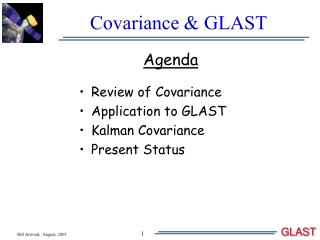# Covariance & GLAST - PowerPoint PPT PresentationDownload PresentationCovariance & GLAST

Covariance & GLAST
Download Presentation## Covariance & GLAST

- - - - - - - - - - - - - - - - - - - - - - - - - - - E N D - - - - - - - - - - - - - - - - - - - - - - - - - - -
##### Presentation Transcript

1. Agenda Review of Covariance Application to GLAST Kalman Covariance Present Status Covariance & GLAST

2. b a ns Review of Covariance Ellipse Take a circle – scale the x & y axis: Rotate by q : Results: Rotations mix x & y. Major & minor axis plus rotation angle q complete description. Error Ellipse described by Covariance Matrix: Distance between a point with an error and another point measured in s’s: where and Simply weighting the distance by 1/s2 and

3. Where I take without loss of generality. Review 2 Multiplying it out gives: This is the equation of an ellipse! Specifically for 1 s error ellipse (ns = 1) we identify: and where And the correlation coefficient is defined as: Summary: The inverse of the Covariance Matrix describes an ellipse where the major and minor axis and the rotation angle map directly onto its components!

4. Review 3 Let the fun begin! To disentangle the two descriptions consider where r = a/b thus q = 0 q = p/2 q = p/4 q = 3p/4 Also det(C) yields (with a little algebra & trig.): Now we’re ready to look at results from GLAST!

5. Covariance Matrix from Kalman Filter Results shown for Binned in cos(q) and log10(EMC) Recall however that KF gives us C in terms of the track slopes Sx and Sy. AxisAsym grows like 1/cos2(q) Peak amplitude ~ .4

6. Relationship between Slopes and Angles For functions of the estimated variables the usual prescriptions is: when the errors are uncorrelated. For correlated errors this becomes and reduces to the uncorrelated case when The functions of interest here are: and A bit of math then shows that: and

7. Angle Errors from GLAST sfhas a divergence at q = 0. However sfsin(q) cures this. sqdecreases as cos2(q) - while sfsin(q) decreases as We also expect the components of the covariance matrix to increase as due to the dominance of multiple scattering. Plot measured residuals in terms of Fit s's (e.g. ) cos(q) log10(EMC)

8. Angle Errors 2 What's RIGHT: 1) cos(q) dependence 2) Energy dependence in Multiple Scattering dominated range What's WRONG: 1) Overall normalization of estimated errors (sFIT) - off by a factor of ~ 2.3!!! 2) Energy dependence as measurement errors begin to dominate - discrepancy goes away(?) Both of these correlate with with the fact that the fitted c2's are much larger then 1 at low energy (expected?). How well does the Kalman Fit PSF model the event to event PSF?

9. Angle Errors 3 Comparison of Event-by-Event PSF vs FIT Parameter PSF (Both Energy Compensated) Difficult to assess level of correlation - probably not zero - approximately same factor of 2.3

10. Angle Errors: Conclusions 1) Analysis of covariance matrix gives format for modeling instrument response 2) Predictive power of Kalman Fit? - Factor of 2.3 - May prove a good handle for CT tree determination of "Best PSF"

11. Present Analysis Status

12. Present Status 2: BGE Rejection Events left after Good-Energy Selection: 1904 Events left in VTX Classes: 26 Events left in 1Tkr Classes: 1878 CT BGE Rejection factors obtained: 20:1 (1Tkr) 2:1 (VTX) NEED FACTOR OF 10X EVENTS BEFORE PROGRESS CAN BE MADE!